• ## 用matlab求二重积分

万次阅读 2020-03-14 00:15:54
matlab求二重积分 题目如下： 已知 ，其中D是由圆周 及坐标轴所围成的第一象限内的闭区域 法一： 积分区域用不等式表示为 二重积分可化为二次积分 matlab中输入命令 syms x y int(int(sqrt(1-x^2-y^2/1+x^2+y^...
2020/3/14
用matlab求二重积分
题目如下：
求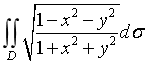，其中D是由圆周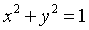及坐标轴所围成的第一象限内的闭区域
法一：
积分区域用不等式表示为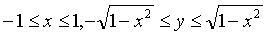二重积分可化为二次积分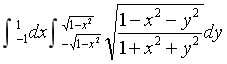matlab中输入命令
syms x y
int(int(sqrt(1-x^2-y^2/1+x^2+y^2),y,-sqrt(1-x^2),sqrt(1-x^2)))

得到
ans =

asin(x) + x*(1 - x^2)^(1/2)

再积分第二层
>> int(ans,x,-1,1)

ans =
0

法二：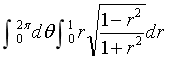对应的matlab命令
 syms theta ; int(ans,theta,0,2*pi)

结果
ans =

(pi*(pi - 2))/2

这里出错在于 没有看是第一象限 应该把2pi改为0.5pi 因此前面也错了，定义域出错
>> int(sqrt(1-x^2-y^2/1+x^2+y^2),y,0,sqrt(1-x^2))

ans =

(1 - x^2)^(1/2)

>>   int((1 - x^2)^(1/2),x,0,1)

ans =

pi/4

至于为什么这里结果是pi/4,还是错就不追究了，说明极坐标的方法更简便
参考来源：(https://jingyan.baidu.com/album/4f7d5712e2de2b1a2019272c.html?picindex=3) 百度文库
展开全文抽象代数
• 今天做matlab数学实验，二重积分（非矩形区域）让我搞了半天，我好菜呀！！！ 好在现在解决了，在这记录一下，如果有朋友遇到相似问题，希望我的记录可以帮助到你。。。 吼吼吼 。开始 求解方法 题目 被积区间...
开头
今天做matlab数学实验，求个二重积分（非矩形区域）让我搞了半天，我好菜呀！！！ 好在现在解决了，在这记录一下，如果有朋友遇到相似问题，希望我的记录可以帮助到你。。。 吼吼吼 。开始
求解方法
题目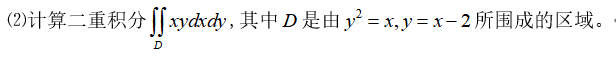被积区间图像 画图代码：
clc,clear all
syms x y;
ezplot('y^2-x',[0,8,-4,4]);
hold on;
ezplot('x-2',[0,8,-4,4]);
hold off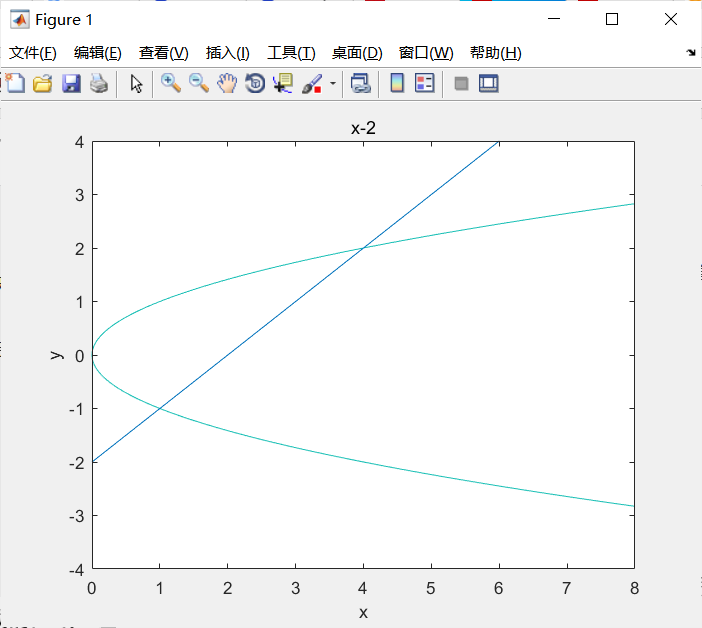然后就要求解了。 求解 第一步先求交点：
[x1,y1]=solve('y^2-x=0','x-2=y');

得到如下结果：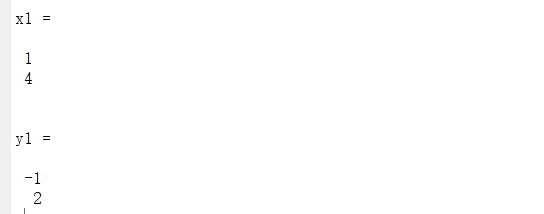然后就可以求积分了。 求二重积分我们通常使用dblquad()函数。 dblquad(‘f’,xmin,xmax,ymin,ymax) 这时我们输入如下代码：
syms x y;dblquad('x*y',y^2,y+2,-1,2)

结果matlab会报错。 这是因为dblquad()只能进行矩形区域的积分。于是我们想到可以将构造如下函数：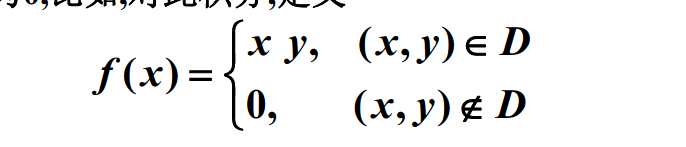由此我第一次写出了如下错误代码：
f(x,y)=x*y.*(x>y^2&x<(y+2));

然而这样并不行，就这样。。。 one hundred years later…
终于我找到了另一种正确办法：
dblquad(@(x,y) x*y.*(x>=y^2&x<=y+2),1,4,-1,2)

这样就可以了。。哈哈，我好智障。 希望，对你有所帮助。
展开全文• matlab二重积分代码Mu-Diff：用于解决多个散射问题的MATLAB工具箱 描述 多重散射是一个非常复杂的波问题，在物理和工程学的许多领域（声学，电磁学，光学，纳米光子学，弹性...）都有广泛的应用。 μ-diff或mu-diff...
•有问必答
• 基于MATLAB二重积分计算方法，数学建模有关，计算科学领域
• 二重积分 %% 目标图像 clc;clear all; x = 0:0.01:pi; % x的范围是0-pi，以0.01为一个小分割点细分出一个范围 y1 = sin(x); % sinx y2 = exp(x); % e^x figure; % 弄一个figure的框体 hold on; % 保持这个窗体，...
二重积分
%% 目标图像
clc;clear all;
x = 0:0.01:pi;  % x的范围是0-pi，以0.01为一个小分割点细分出一个范围
y1 = sin(x);    % sinx
y2 = exp(x);    % e^x

figure;         % 弄一个figure的框体
hold on;        % 保持这个窗体，保证后面的内容都画在了上面，hold off解除

subplot(221);   % 开辟子窗体，221:共2行2列，里面的第一个
fill(x,y1,'r'); % 画图并填充颜色，变量是x，函数是y1，颜色是r，红色
title('y=sinx');% 设置标题，必需先fill，才能添加标题，否则会失败

subplot(222);   % 开辟子窗体，222，共2行2列，里面第二个
fill(x,y2,'b'); % b 蓝色
title('y=e^x');

subplot(223);   % 开辟子窗体，223，共2行2列，里面第三个
fill([x, fliplr(x)],[y1,fliplr(y2)],'g');
% 两个函数直接填充颜色，每一个方括号代表一个函数的内容
title('两个函数中间的部分');
hold off;
%% 对上面的函数进行计算定积分
syms x;
f=exp(x) - sin(x);
res_int = int(f, x, 0, pi);
disp(res_int);
%% 计算二重积分
clear all;
f = @(x,y)x.*cos(y); % 定义一个函数句柄，两个变量分别是x,y
% 这样定义函数句柄时，需要在所有的运算前加一个.（表示对应元素各自计算，不按照矩阵的规则）
ymax = @(x) x;  % 根据0<=y<=x处，右方的y=x反解出y，
res = integral2(f,0,1,0,ymax);
% 计算二重积分的函数
% integral2(fun, xmin, xmax, ymin, ymax)
disp(res);
%%曲面
[x,y] = meshgrid(0:0.001:1, 0:0.001:1);
% 设置x和y的区间，以及细分程度
z = (x.*cos(y));  % 使用一个z来代表这个函数
mesh(x,y,z)  % 画出二维曲面图像
title('x*cos(y)')

展开全文• 凯鲁嘎吉 - 博客园 ... 定积分解决的是一维连续量求和的问题，而解决多维连续量的求和问题就要用到重积分了。...正是因为重积分从计算上来说仍是使用的定积分的方法，MATLAB系统并没有提供专门的命令...

凯鲁嘎吉 - 博客园
http://www.cnblogs.com/kailugaji/
定积分解决的是一维连续量求和的问题，而解决多维连续量的求和问题就要用到重积分了。重积分是建立在定积分的基础上的，它的基本思想也是将重积分化为定积分来计算，其中关键是积分限的确定，这也是重积分的难点所在。正是因为重积分从计算上来说仍是使用的定积分的方法，MATLAB系统并没有提供专门的命令函数来处理重积分，因此在我们确定了积分限后仍是使用int()命令来处理重积分问题。有些积分区间形状比较复杂，为了方便表达积分的上下限常常把比较复杂的区间分割成若干个相对简单的区间，然后对不同的区间分别积分，最后把各个积分结果相加起来。
计算其中，wrr.m:

function tt=wrr(r,v,c,b,y,w)
syms u t
p=-(r/c)^v;
g=exp(p);
h=(b/y)*(t/y)^(b-1);
hu=(b/y)*(u/y)^(b-1);
f=int(g*hu,u,0,t);
tt=int(t*g*h*exp(-f),t,0,w);
tt=vpa(tt,10);

//结果为：
>> clear
>> tt=wrr(0.5,0.787,0.033,1.097,0.241,2)

tt =

0.002183207532


对于二元函数的符号积分，可以先转化成逐次积分形式，利用int函数进行求解。

转载于:https://www.cnblogs.com/kailugaji/p/8280736.html
展开全文• 自编Python程序实现数值计算矩形区域二重积分，使用复化辛普森法。以函数f=xsiny在0和pi/2区域上的积分为例。网格节点数m，n需为2的倍数。对于非矩形区域可以使用虚拟节点和区域，填补为矩形区域后计算，填补区域上...
• 蒙特卡洛方法实现三重积分与二重积分，北邮科学计算与编程作业
• 佛山科学技术学院 实 验 报 告 课程名称 数值分析 实验项目 ... 2学会复合梯形复合Simpson和龙贝格积分公式的编程与应用 3探索二重积分在矩形区域的数值积分方法 二实验要求 按照题目要求完成实验内容 写出相应的M
• %函数定义处end%%monte_carlo.m%蒙特卡洛计算二重积分function result=monte_carlo(a,b,c,d,n,m)%先y后x积分，a是x积分下限，b是x积分上限，c是y积分下限，d是y积分上限，n，m是蒙特卡洛参数sumxff=0...
• MATLAB的数值积分工具箱 用龙贝格积分法计算积分的程序，matlab语言编写，希望能对大家有用
• matlab二重积分代码目录 DIRECTFN软件包是免费软件，用于准确有效地评估在符合三角形或四边形网格的情况下，由Galerkin MoM表面积分方程公式产生的4-D奇异积分。 DIRECTFN的全数值算法适用于以下应用： 弱而强烈的...
• 在第本系列第六篇中，我们讲解了一下相对来讲最普通的不定积分和定积分的问题，相信大家对积分已经有了大概的了解了，可是仍有一些小问题，比如如何计算多个变量的积分，比如二重积分、甚至三重三重积分呢？...matlab matlab积分 matlab反解函数
• ## Matlab求解定积分/不定积分

万次阅读 多人点赞 2019-10-03 14:30:49
符号积分函数：int 格式：int(f,x,a,b) 功能：计算定积分 格式：int(f,x) 功能：计算不定积分 使用int函数之前，先用syms声明x是符号变量 例： 代码： syms x y1=1/(1+x^4); y2=(x*exp(x))/(1+x)^2; y3=...
• 基于MATLAB的蒙特卡罗方法求解二重积分.pdf
• 计算如下不定二重积分 实验程序： syms x y f=(x*exp(-x*y))//进行二次简单的不定积分，分别进行计算，计算二次就好了 c=int(f,'x') int(c,'y') 实验结果： y=dsolve('D2y+4*Dy+29*y=0','y(0)=0,Dy(0)=...
• 复化辛普森公式求二重积分matlab源码 直接拷贝到matlab编辑器，傻瓜式操作。具体算法自行探究，网上都有，小编只提供代码。用的好的请加个关注，篱落～～成殇～～再次先行谢过。 %%%%%%%%%% 2020.6.5 %%%%%%%%% %%%%...
• MATLAB求解无穷区间定积分 部分源码 clear;clc;close all k=1; a=-10; %积分下限 b=10; %积分上限 global x
• MATLAB中进行二重积分的函数integral2和quad2d q = integral2(fun,xmin,xmax,ymin,ymax) q = integral2(fun,xmin,xmax,ymin,ymax,Name,Value) integral2 Numerically evaluate double integral. Q = integral2...复杂函数积分
• 复化梯形公式求二重积分matlab源码 %%%%%%%%%% 2020.6.5 %%%%%%%%% %%%%%%%%%%复化梯形公式求二重积分%%%%%%%%%%%%%%%%%%% Liu Deping %%%%%%%%%clear all;%%%被积函数及积分上下限导入；s=input('请输入函数表达式......

# matlab求二重积分matlab 订阅